JEE  >  DC Pandey Solutions: Refraction of Light- 1

# DC Pandey Solutions: Refraction of Light- 1 | DC Pandey Solutions for JEE Physics

 1 Crore+ students have signed up on EduRev. Have you?

Introductory Exercise 28.1

Ques 1: There is a dust particle on a glass slab of thickness t and refractive index μ = 1.5. When seen from one side of the slab, the dust particle appears at a distance 6 cm. From other side it appears at 4 cm. Find the thickness t of the glass slab.
Sol:
Actual distance from one side = μ - times = 6 × 1.5 = 9 cm
From other side = 4 × 1.5 = 6 cm
∴ Total thickness = (9 + 6) cm = 15 cm

Ques 2: Given that 1μ2 = 4/3, 2μ3 = 3/2. Find 1μ3.
Sol:
1μ2 × 2μ3 × 3μ1 = 1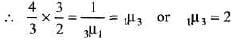Ques 3: A monochromatic light beam of frequency 6.0 × 1014 Hz crosses from air into a transparent material where its wavelength is measured to be 300 nm. What is the index of refraction of the material?
Sol: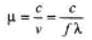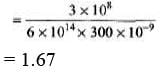Introductory Exercise 28.2

Ques 1: A glass sphere (μ = 1.5) with a radius of 15.0 cm has a tiny air bubble 5 cm above its centre. The sphere is viewed looking down along the extended radius containing the bubble. What is the apparent depth of the bubble below the surface of the sphere?
Sol: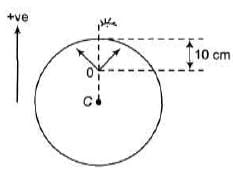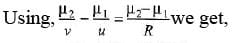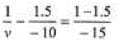Solving we get v = - 8.57 cm

Ques 2: One end of a long glass rod (μ = 1.5) is formed into a convex surface of radius 6.0 cm. An object is positioned in air along the axis of the rod. Find the image positions corresponding to object distances of
(a) 20.0 cm (b) 10.0 cm (c) 3.0 cm from the end of the rod.
Sol: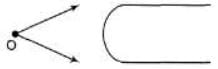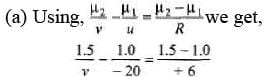Solving we get v = + 45 cm Similarly other parts can be solved.

Ques 3: A dust particle is inside a sphere of refractive index  4/3. If the dust particle is 10.0 cm from the wall of the 15,0 cm radius bowl, where does it appear to an observer outside the bowl.
Sol: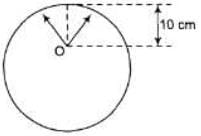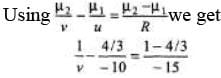Solving we get v = -9.0 cm

Ques 4: A parallel beam of light enters a clear plastic bead 2.50 cm in diameter and index 1.44. At what point beyond the bead are these rays brought to a focus?
Sol: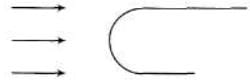Using the equation,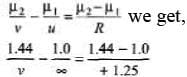∴  v = 4.0cm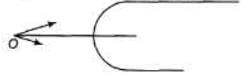Ques 5: The left end of a long glass rod of index 1.6350 is grounded and polished to a convex spherical surface of radius 2.50 cm. A small object is located in the air and on the axis 9.0 cm from the vertex. Find the lateral magnification.
Sol:
Using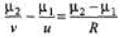we get,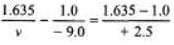v = + 1.4 cm
Now,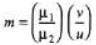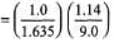= - 0.0777

The document DC Pandey Solutions: Refraction of Light- 1 | DC Pandey Solutions for JEE Physics is a part of the JEE Course DC Pandey Solutions for JEE Physics.
All you need of JEE at this link: JEE

210 docs

## DC Pandey Solutions for JEE Physics

210 docs

Track your progress, build streaks, highlight & save important lessons and more!

,

,

,

,

,

,

,

,

,

,

,

,

,

,

,

,

,

,

,

,

,

;